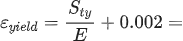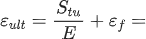﻿ Stress-Strain Curve Calculator | MechaniCalc

The Stress-Strain Curve calculator allows for the calculation of the engineering stress-strain curve of a material using the Ramberg-Osgood equation. See the reference section for details on the methodology and the equations used.

### Material Property Inputs

Enter the material properties in either US or SI units:

### Points for Stress-Strain Curve

The points for the engineering stress-strain curve are shown below:

Display Units:

Cannot display plot -- browser is out of date.
Yield Point Ultimate Point

### Ramberg-Osgood Equation

The stress-strain curve is approximated using the Ramberg-Osgood equation, which calculates the total strain (elastic and plastic) as a function of stress:

where σ is the value of stress, E is the elastic modulus of the material, Sty is the tensile yield strength of the material, and n is the strain hardening exponent of the material which can be calculated based on the provided inputs.

Based on the specified material properties, the value of the strain hardening exponent, n, is:

 n  = strain hardening exponent

### Strain Values at Yield and Ultimate Strength Points

The strain value associated with the yield strength is:Reference Values

 Sty = E =

Note that a plastic strain of 0.002 is assumed. This is consistent with the 0.2% offset method, as described here.

The strain value associated with the ultimate strength is:Reference Values

 Sty = E = εf =

where εf is the plastic strain at failure and is simply the percent elongation expressed in decimal form:

 εf = eL / 100% =

Reference Values

 eL =

## Need More Functionality?

•
• Price
• Create Materials
• Create Cross Sections
• Save Files
• Reporting
• Free
• Limited
• None
• Pre-defined Materials
• Pre-defined Cross Sections
• No Saved Files
• No Reporting
•  USD $9.99 /month USD$99.99 /year
• Full
• Individual
• Create Materials
• Create Cross Sections
• Unlimited Saved Files
• Unlimited Reporting
•  USD $29.99 /month USD$299.99 /year
• Full
• Create Materials
• Create Cross Sections
• Unlimited Saved Files
• Unlimited Reporting
• Bulk pricing (≥20 educational licenses)
• Full